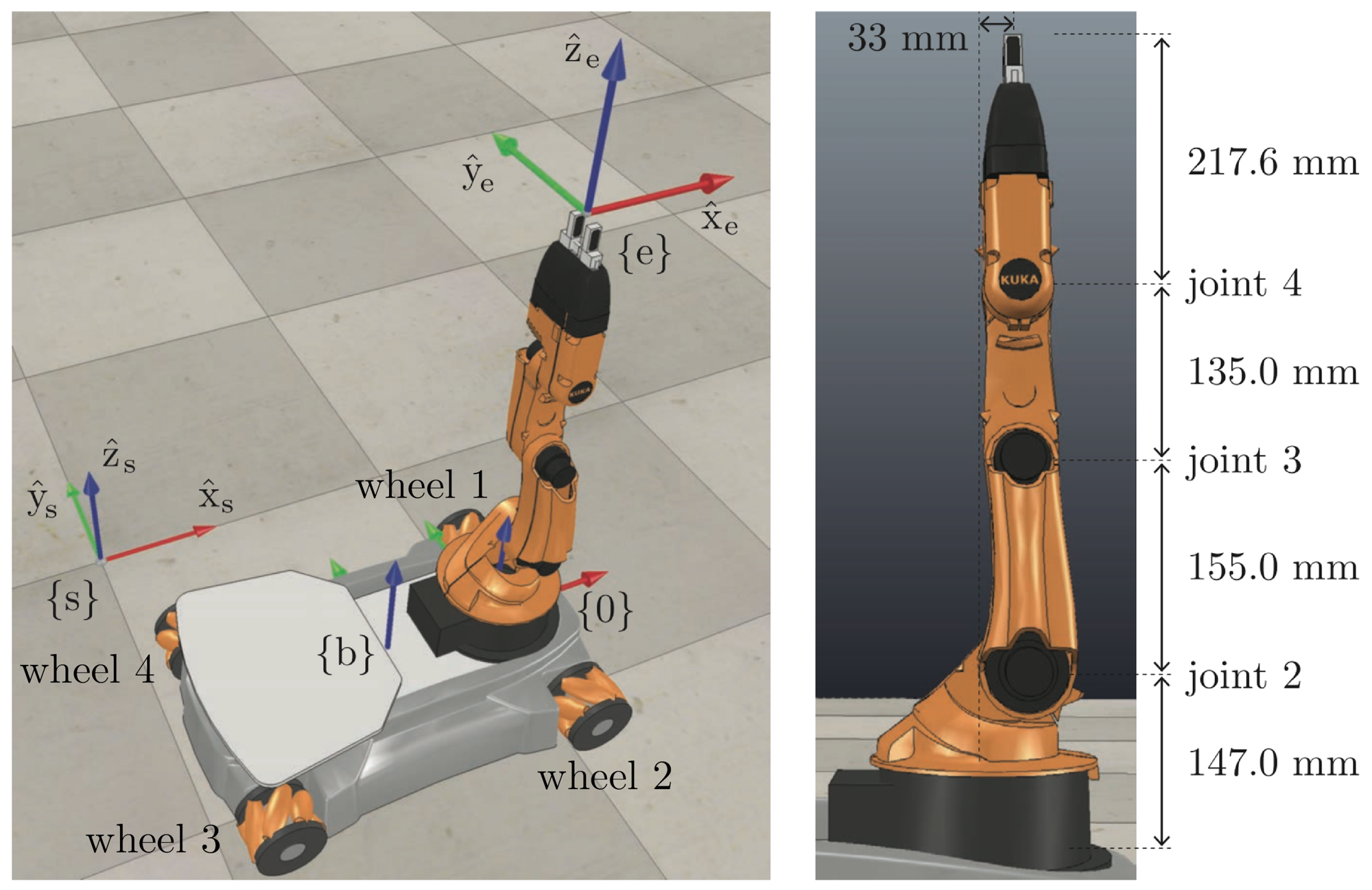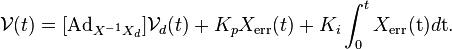# Mobile Manipulator in Simulator

## Project Description

In this project, I wrote a complete software for a mobile manipulation pick-and-place task with a youBot. The task is accomplished by first planning a trajectory for the end-effector, performing odometry calculation for the omnidirectional mobile base, commanding the robot with a feedforward-plus-PI controller. The software is also divided into submodules (classes) according to the task for better organizing and testing. Additionally, I implemented the joint limit to avoid self-collision and singularity. The final outcome is ran by CoppeliaSim for demonstration.## Approach

The entire project includes three main components: Kinematics Simulator, Reference Trajectory Generation, and Feedforward + PID Controller

### youBot Kinematics SimulatorThe state of the youBot consists of x,y, orientation, five arm joint angles, and four wheel angles

$$X = \begin{bmatrix} x & y & \theta_b & \theta_1 & \theta_2 & \theta_3 & \theta_4 & \theta_5 & \phi_1 & \phi_2 & \phi_3 & \phi_4 \end{bmatrix}$$

The control input consists of five arm joint speeds and four wheel speeds. Each element has its own limit range to match the real world behavior.

$$U = \begin{bmatrix} \dot{\theta_1} & \dot{\theta_2} & \dot{\theta_3} & \dot{\theta_4} & \dot{\theta_5} & \dot{u_1} & \dot{u_2} & \dot{u_3} & \dot{u_4} \end{bmatrix}$$

For simplicity, this project uses first-order Euler step for updating the state. It can be replaced by Runge-Kutta, etc.

$$\theta_{t+1} = \theta_{t} + \dot{\theta} \times \delta t \newline \phi_{t+1} = \phi_{t} + \dot{\phi} \times \delta t \newline \newline V_b = F\delta_{\theta} \newline \newline T_{bb’} = e^{[V_b]} \to \delta{q_b} = (\delta{\phi_b},\delta{x_b},\delta{y_b}) \newline \newline q_{t+1} = q_{t} + \delta{q}$$

### Reference Trajectory Generation

The trajectory generation focuses on having the robot joints pass through a series of via points at specific time. The method used in this project is polinomial interpolation.

For each joint X, there are k via points with j = k-1 segments. The joint trajectory for segment j is:

$$\beta(t_j + \delta{t}) = a_{j0} + a_{j1}\delta{t} + a_{j2}\delta{t^2} + a_{j3}\delta{t^3}$$

with four constraints:

$$\beta{(t_j)} = \beta_{j} \newline \dot{\beta{(t_j)}} = \dot{\beta_{j}} \newline \beta(t_j + \delta{t}) = \beta_{j+1} \newline \dot{\beta}(t_j + \delta{t}) = \dot{\beta}_{j+1}$$

Solve for all the a in the equation to obtain the polinomial interpolation.

### Feedforward + PID Controller

The commanded twist is calculated with the following equation:The error twist is:

$$X_{err} = log(X^{-1}X_{d})$$

The feedforward reference twist is:

$$V_d = \frac{1}{\delta{t}}log({X_d}^{-1} X_{d,next})$$

## Steps

1. Initialize youBot kinematics
a. Omni-directional mobile base
b. 5-DoF Arm
2. Generate trajectory for the end-effector of the arm
3. Start simulation:
a. Calculate current state
b. Calculate control force for both joints and wheels using feedforward plus PID
c. Check joint limit to avoid self-collision and singularity
d. Update odometry using Euler Method
e. Repeat
4. Plot

## Results

Here is an example demo with the error plot## Source Code

Unfortunately, the source code cannot be shared due to the copyright of this project. If you are interested, please feel free to contact me.

## Reference

All images and equations comes from Modern Robotics: Mechanics, Planning, and Control (Kevin Lynch and Frank Park, Cambridge University Press 2017)

Updated: